Successfully reported this slideshow.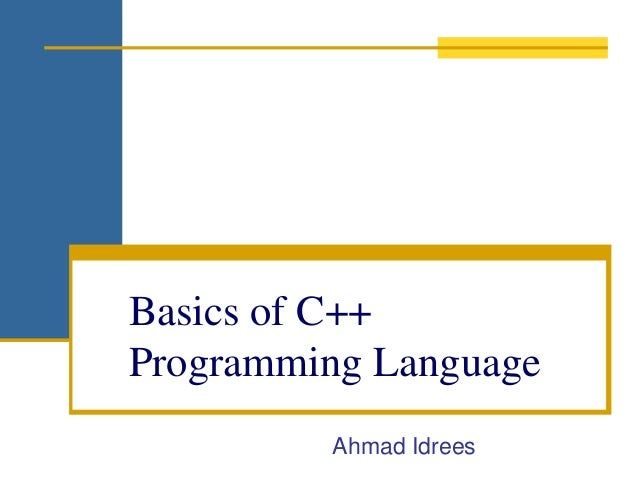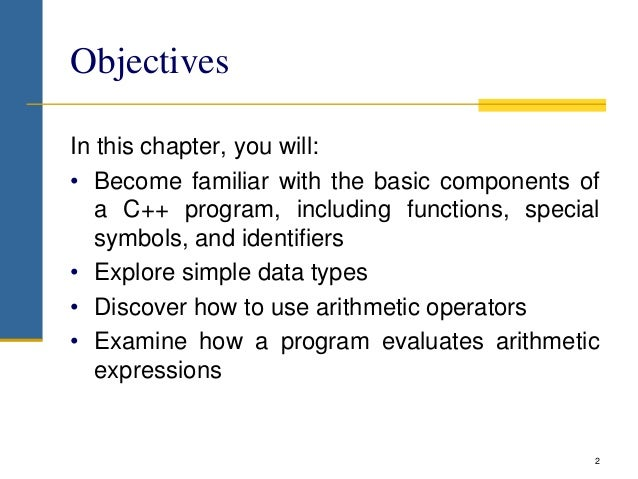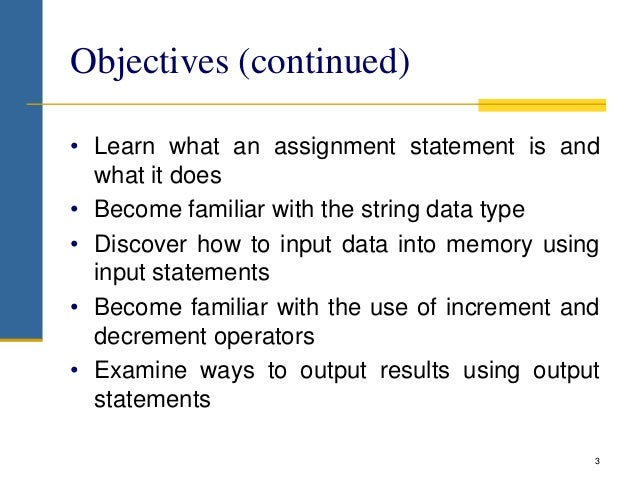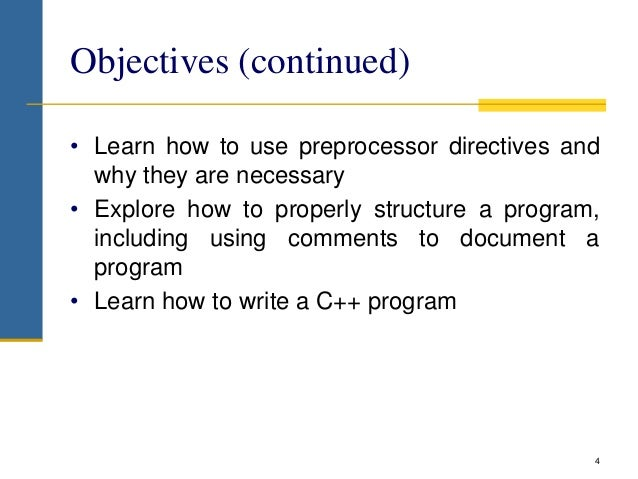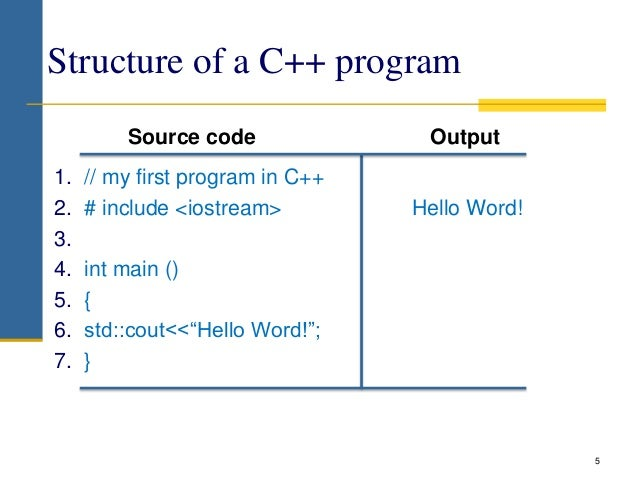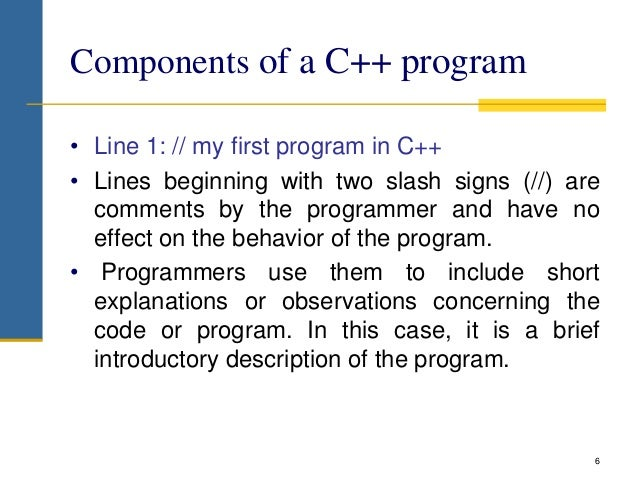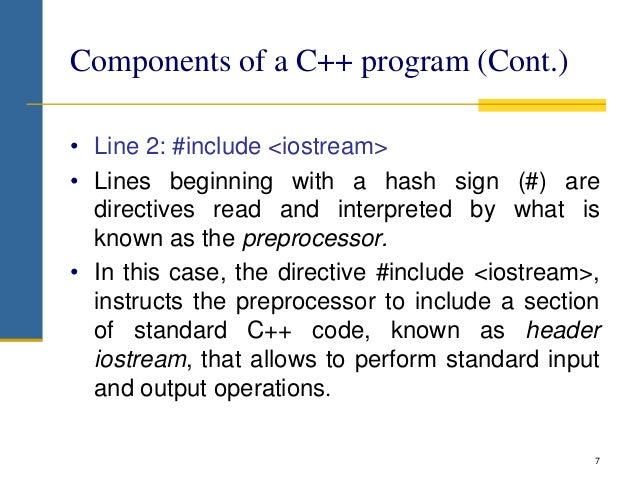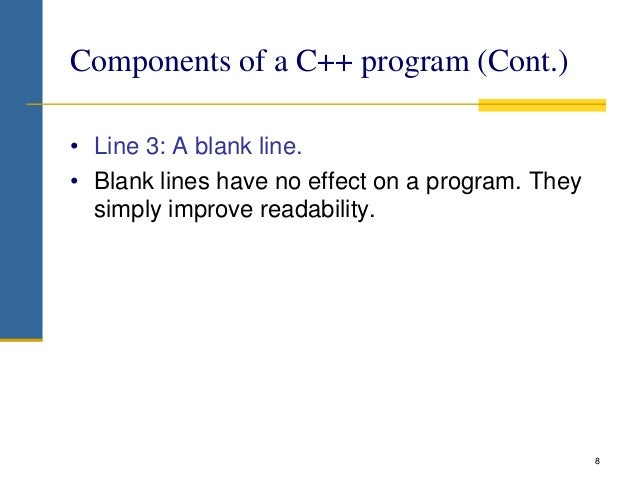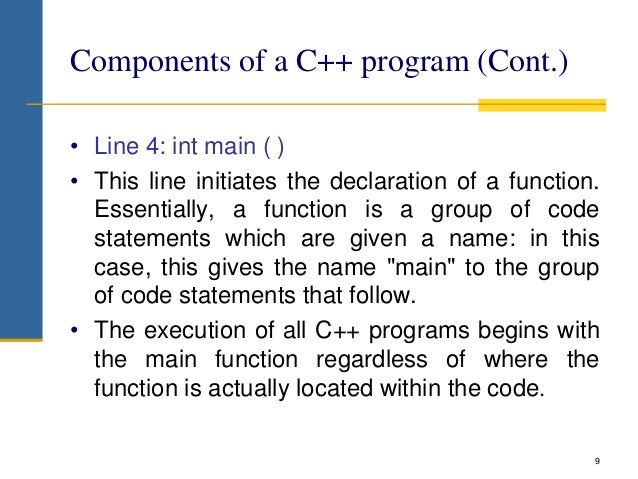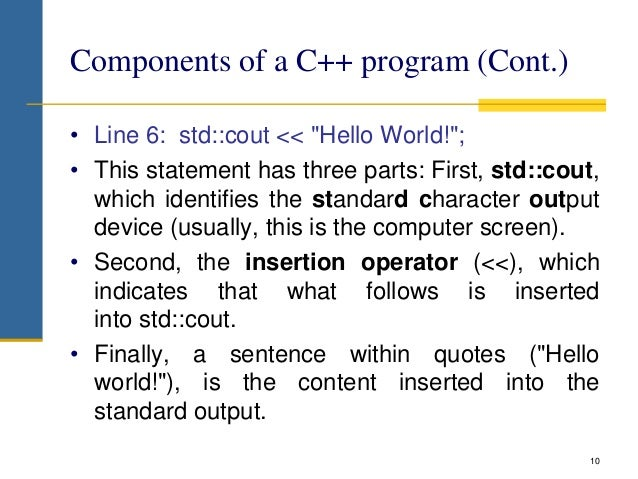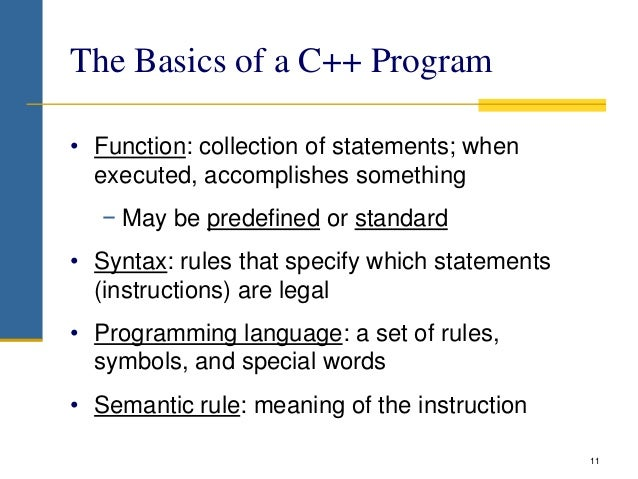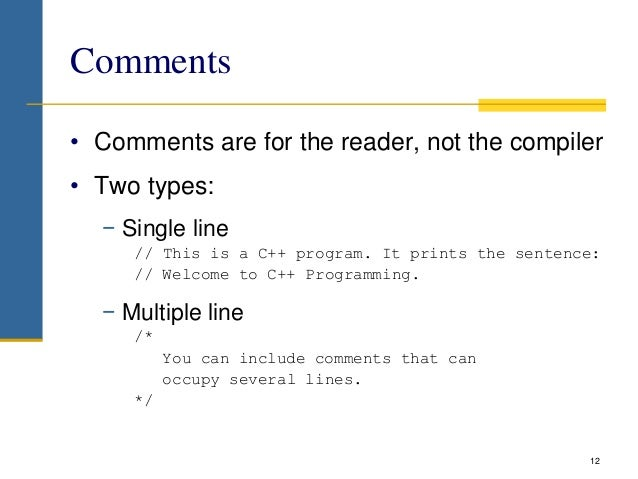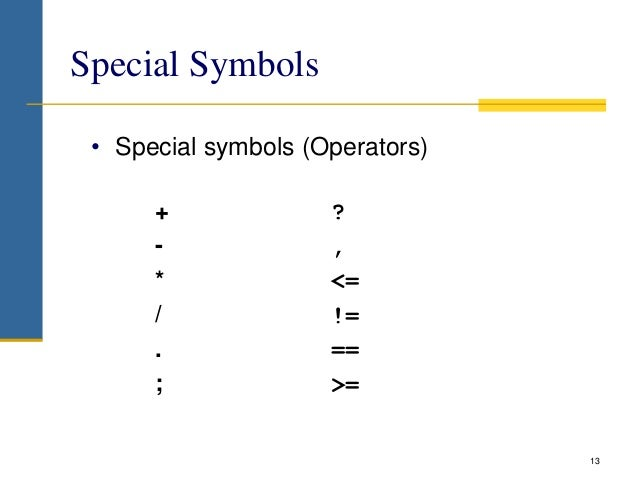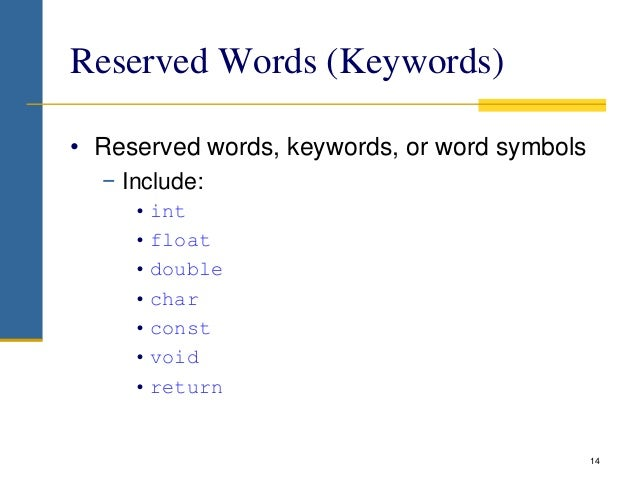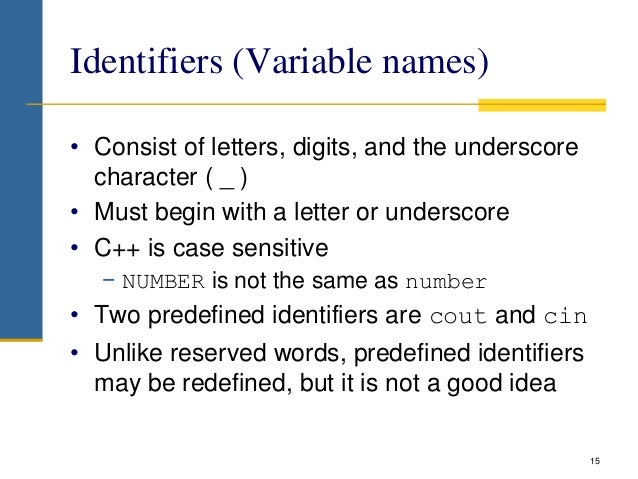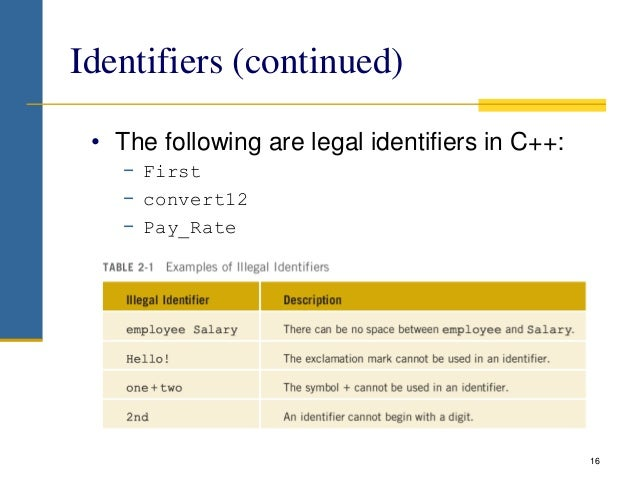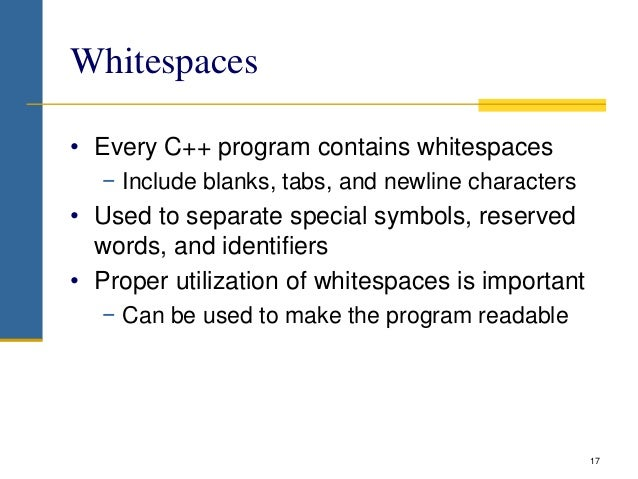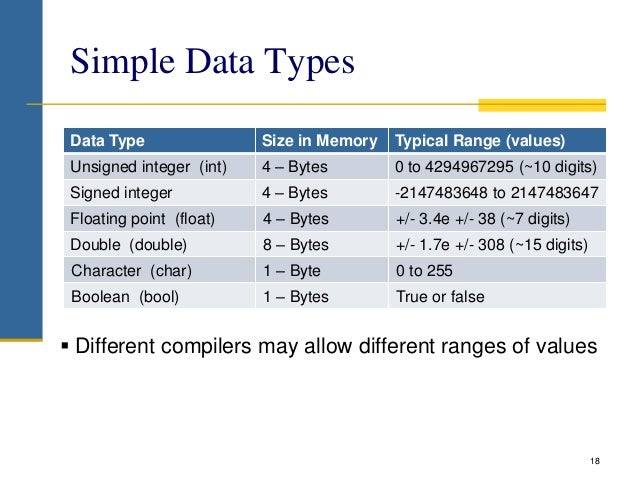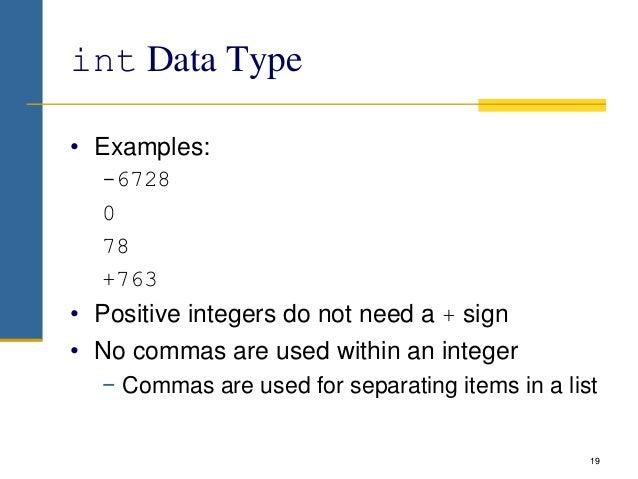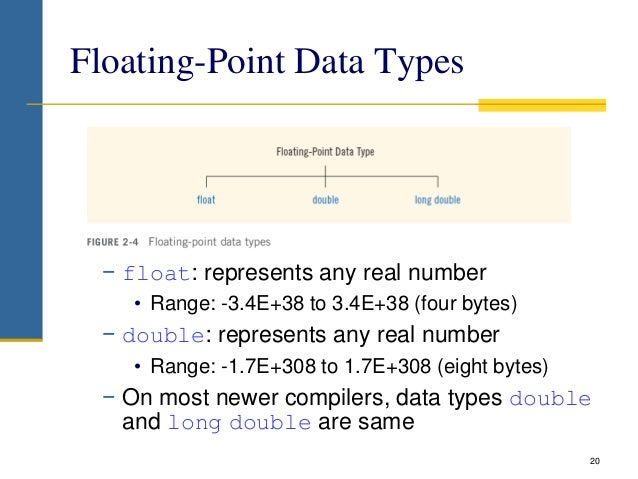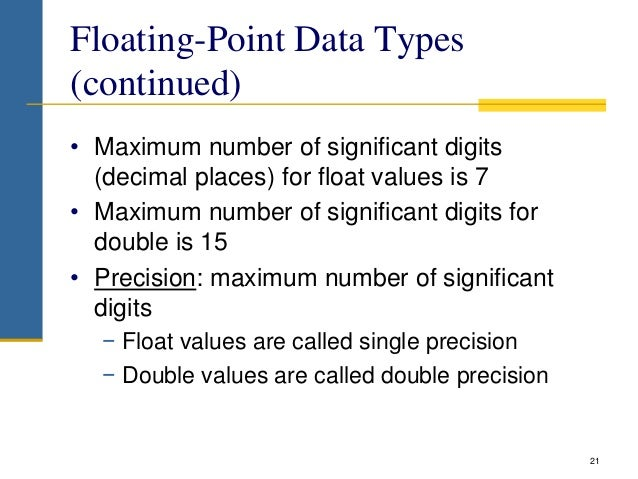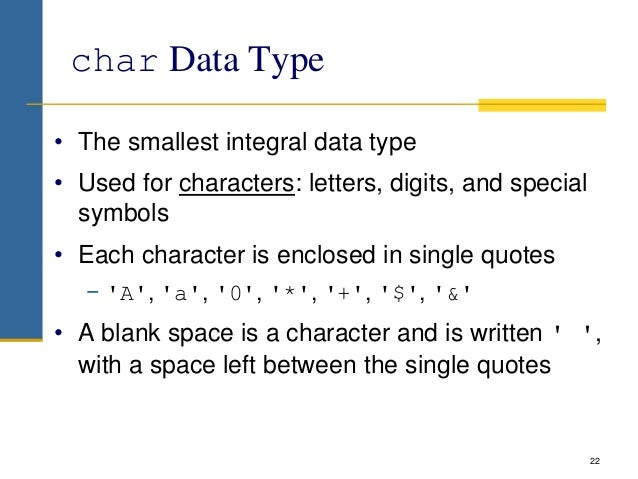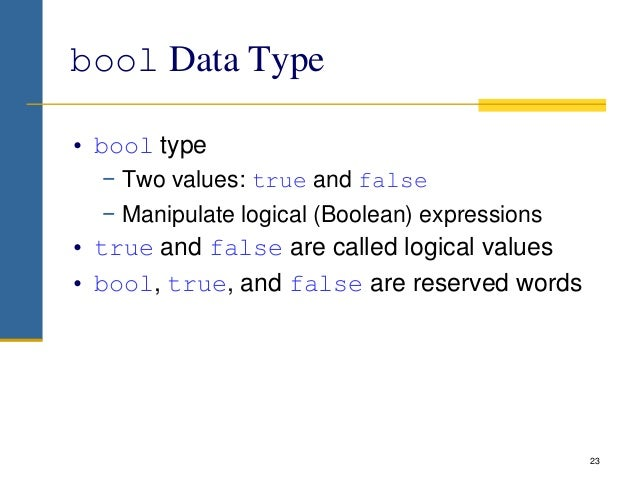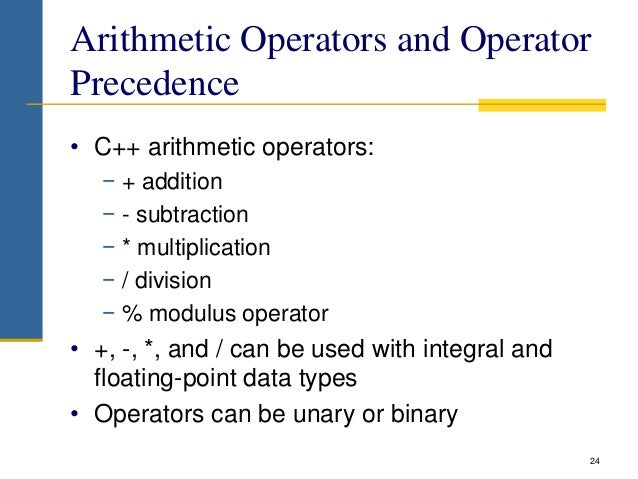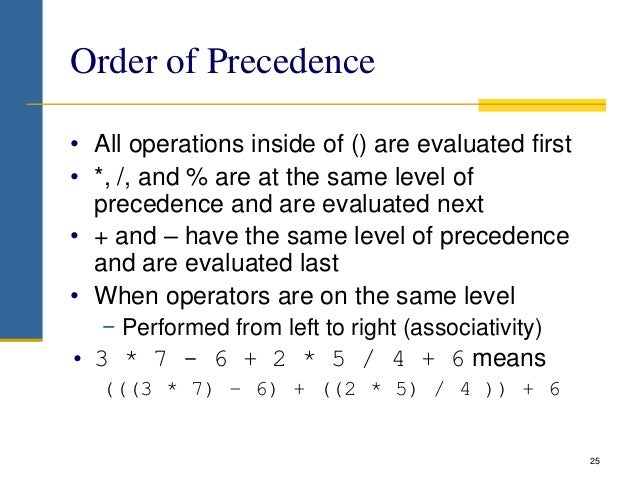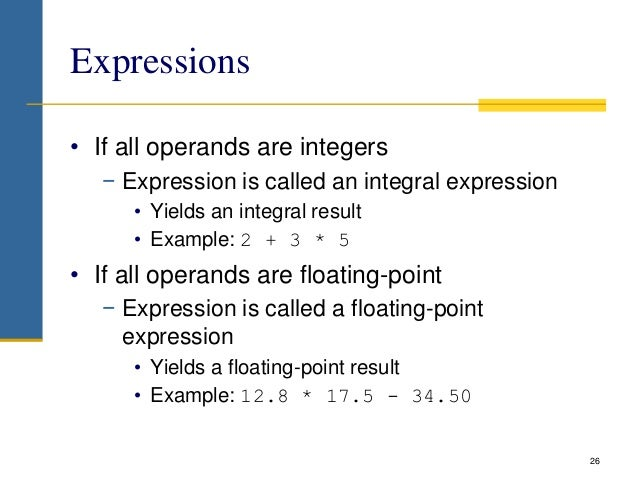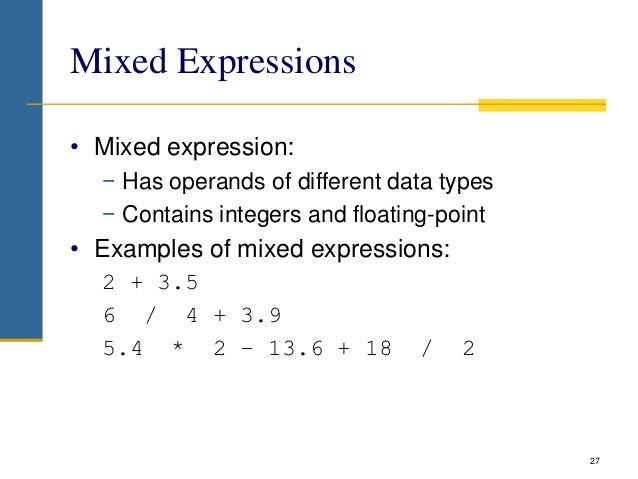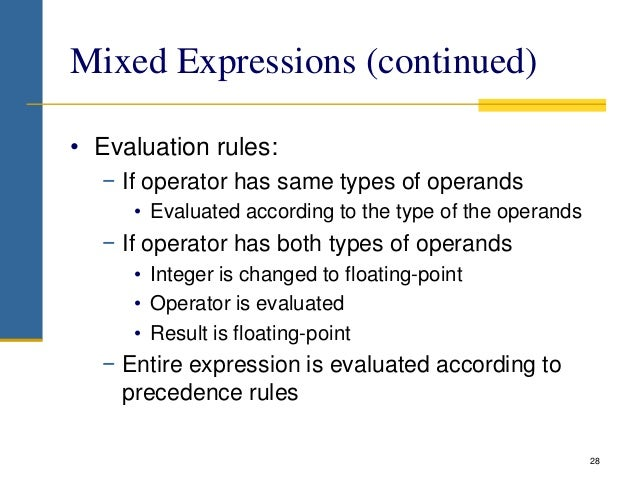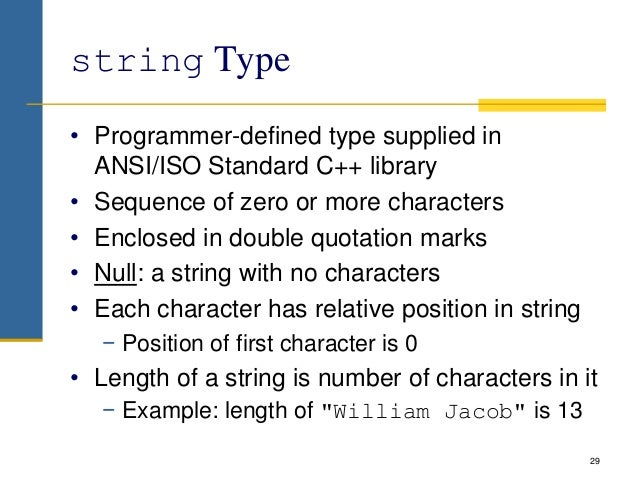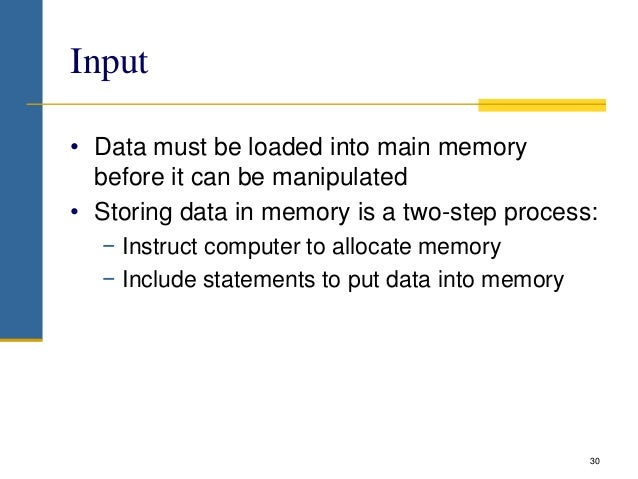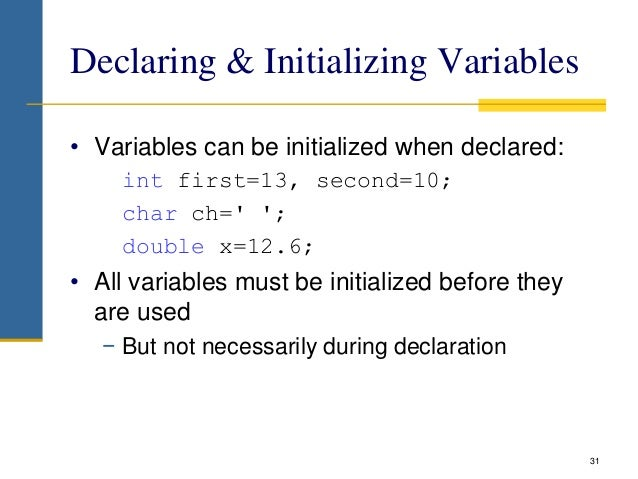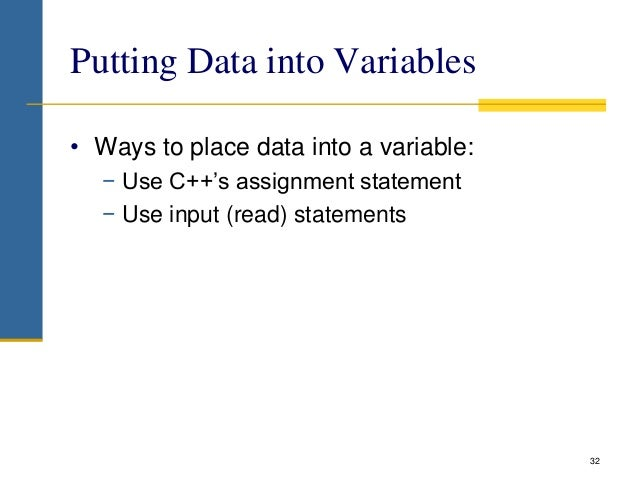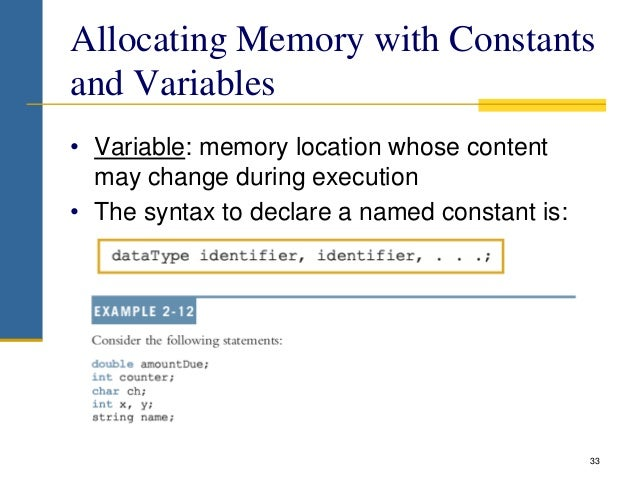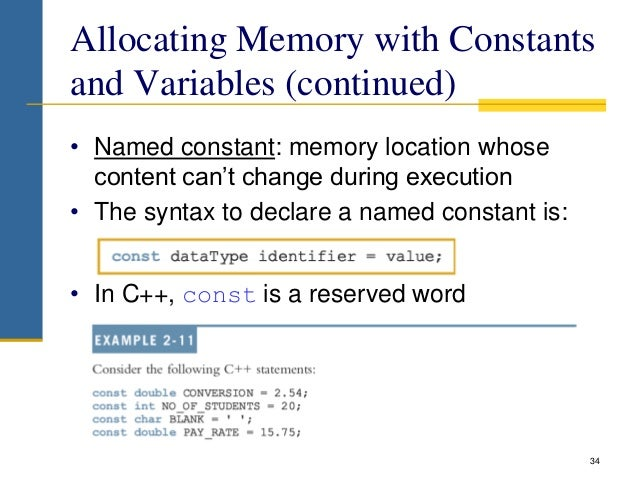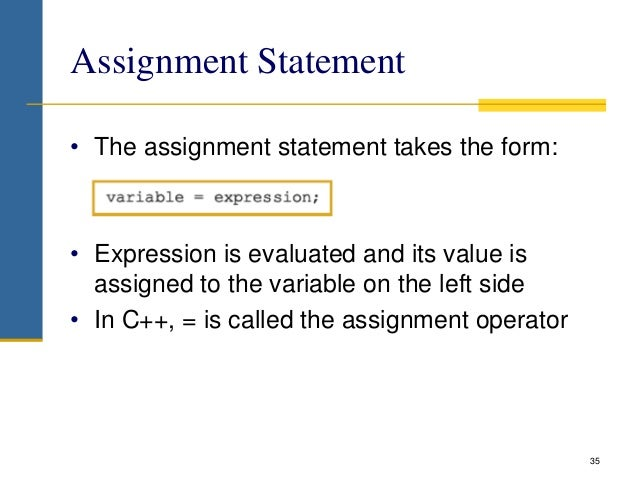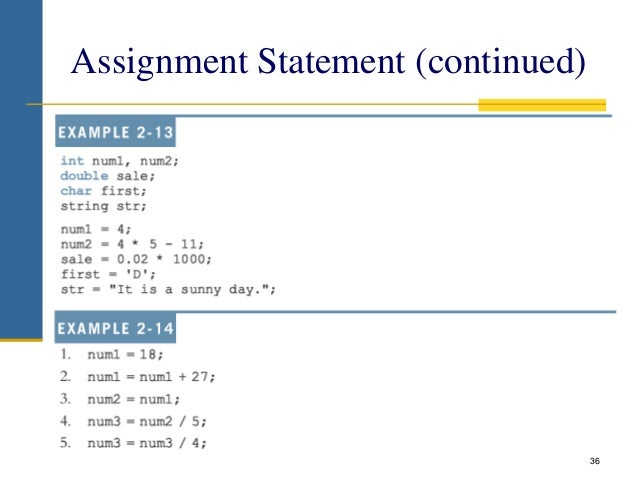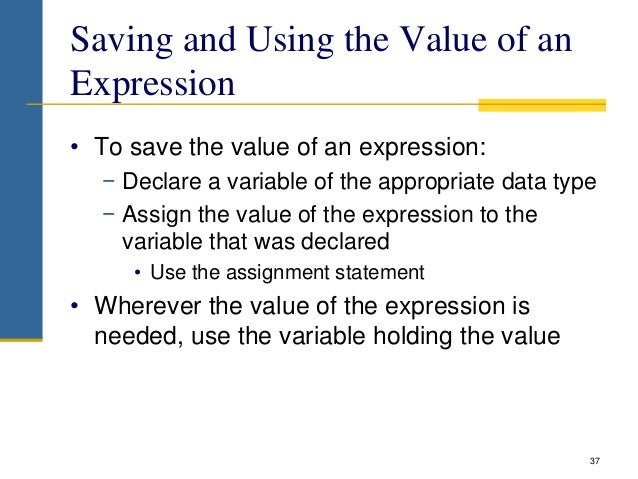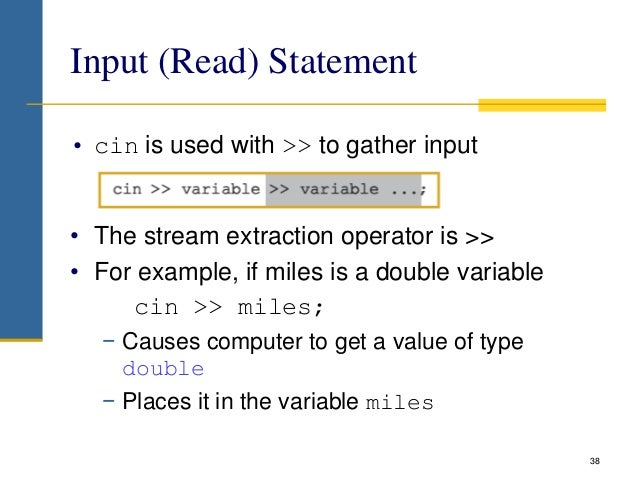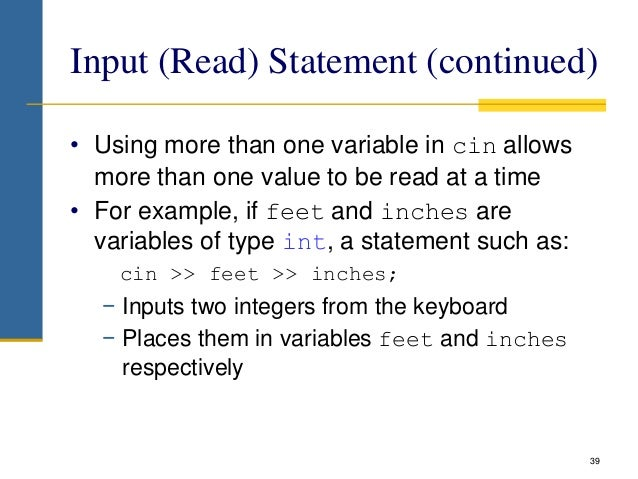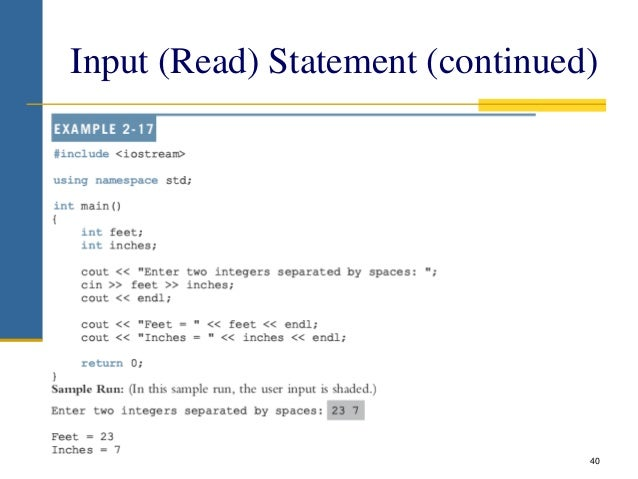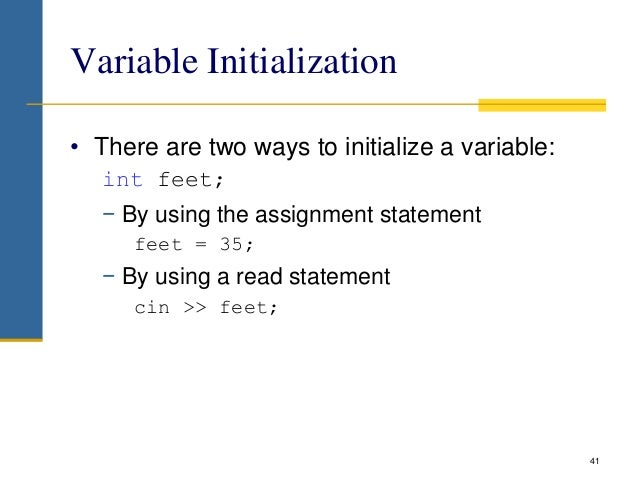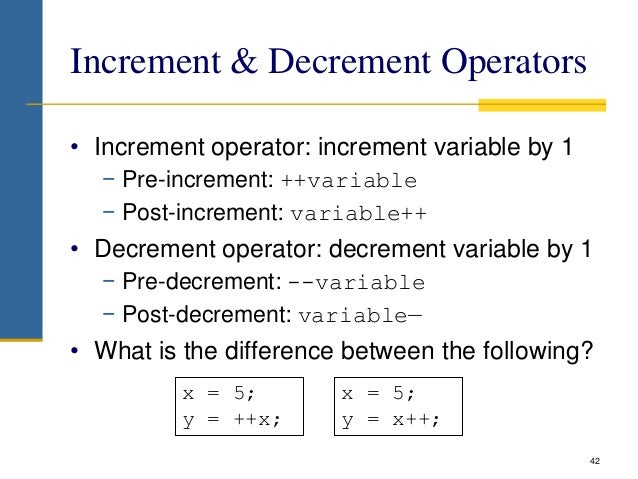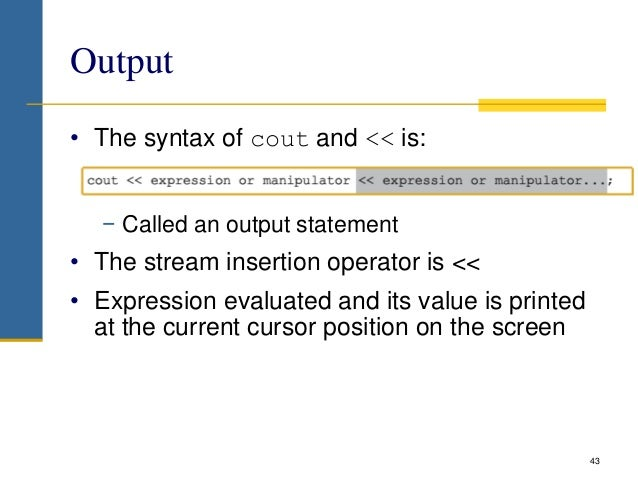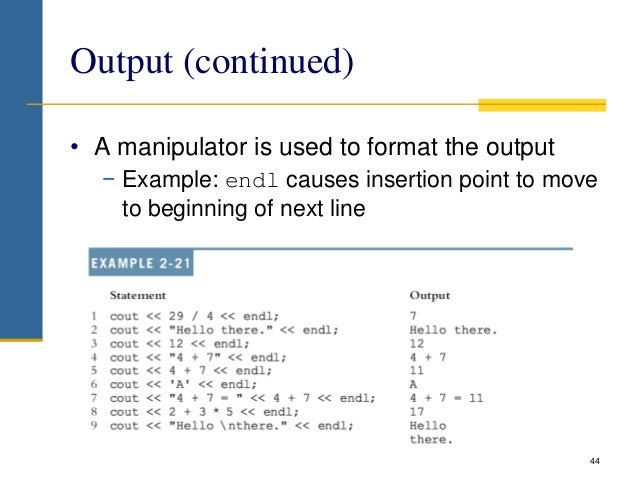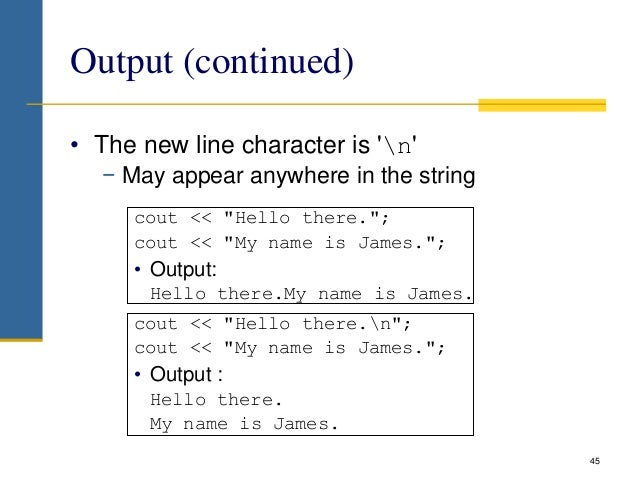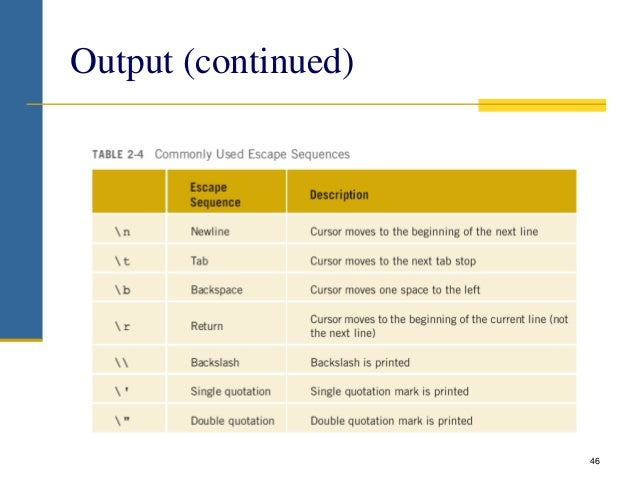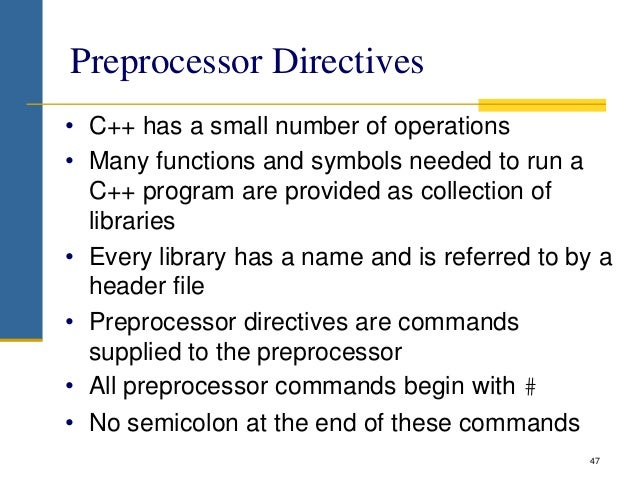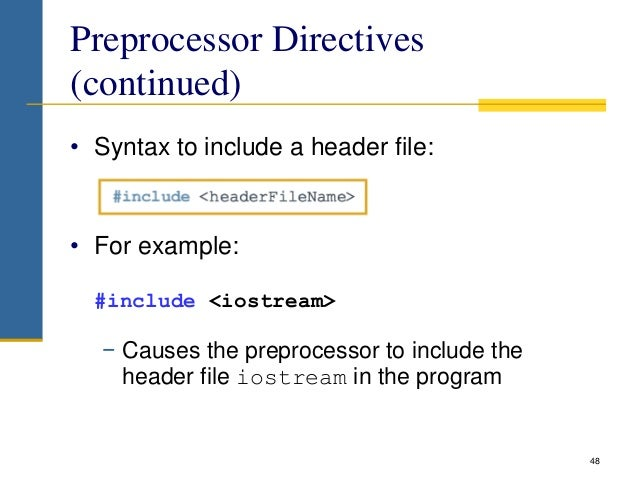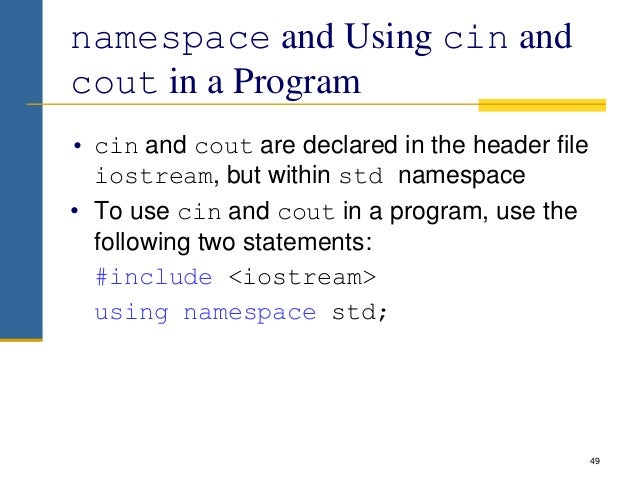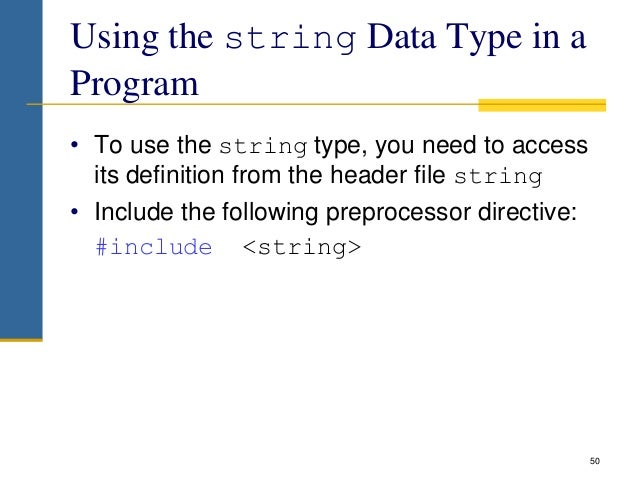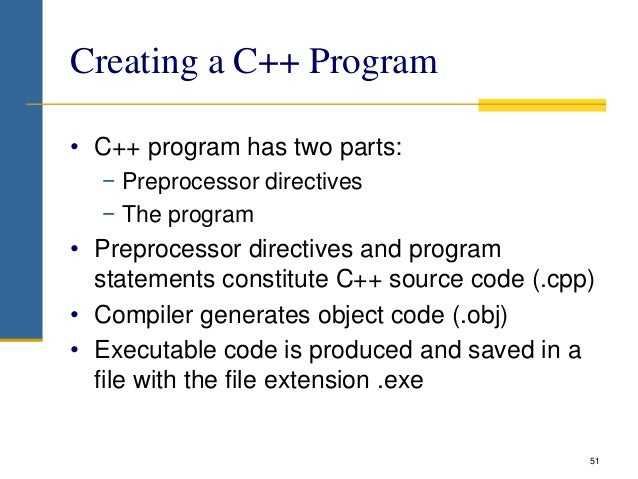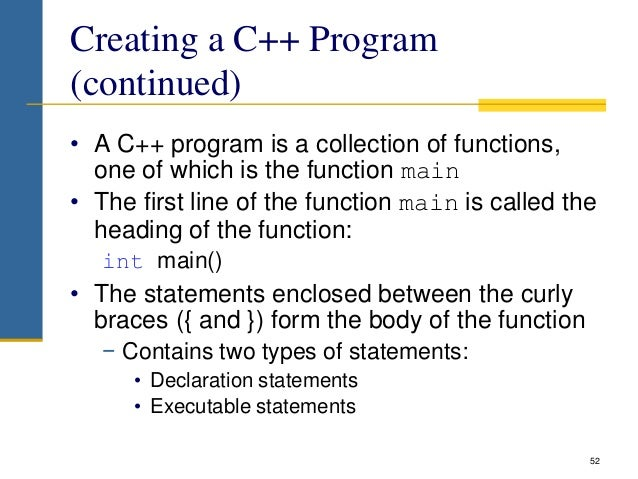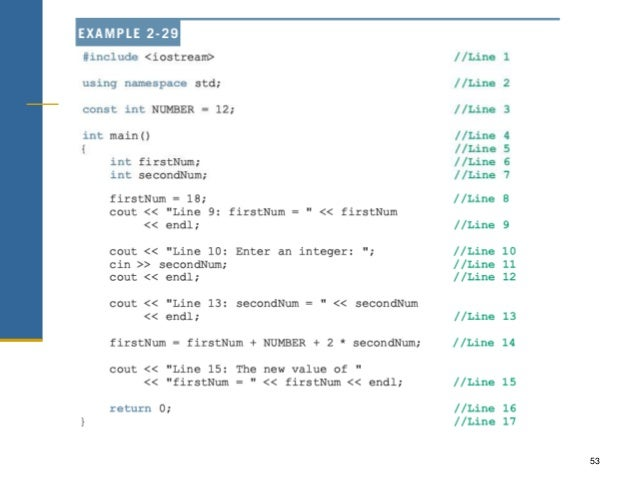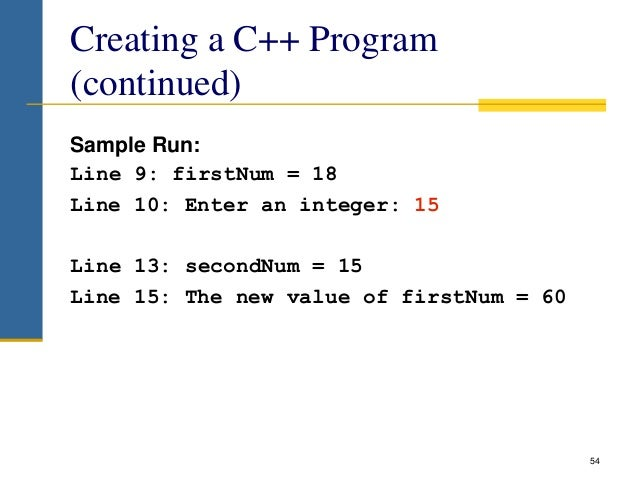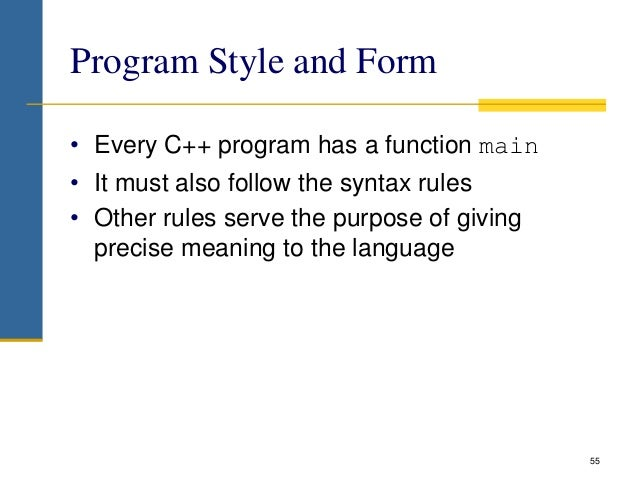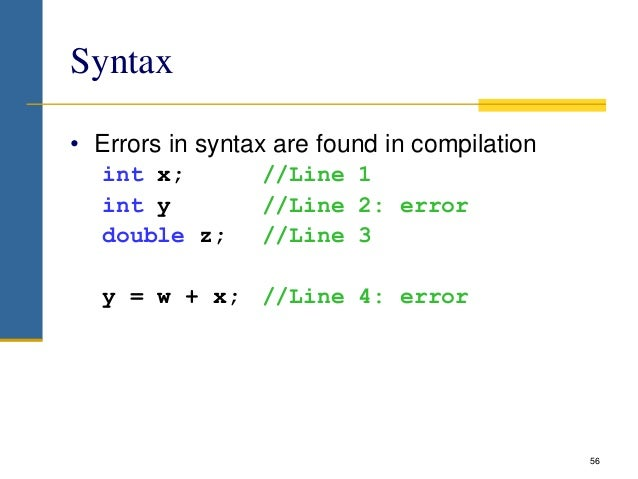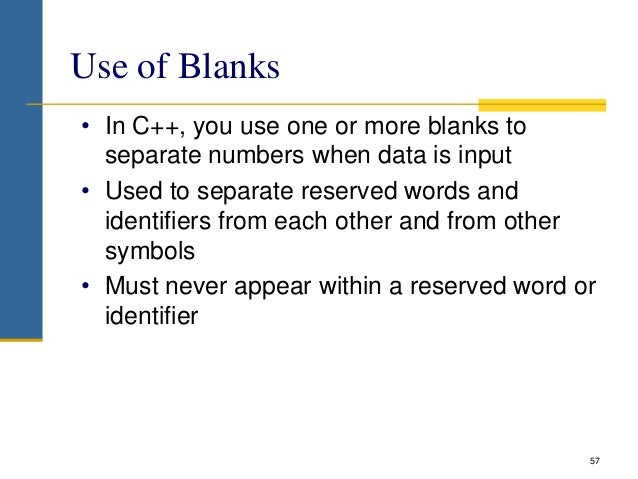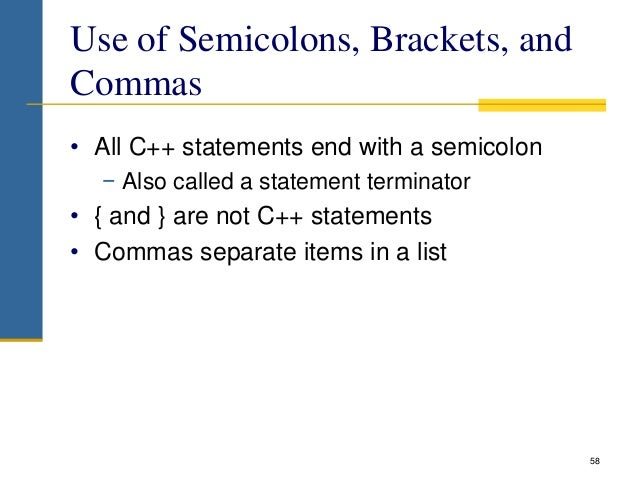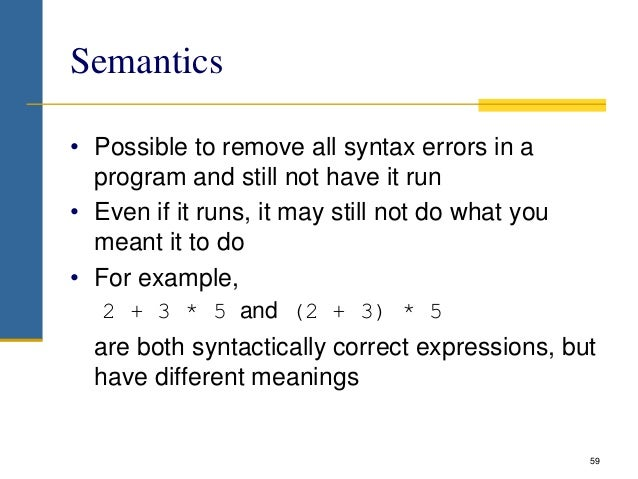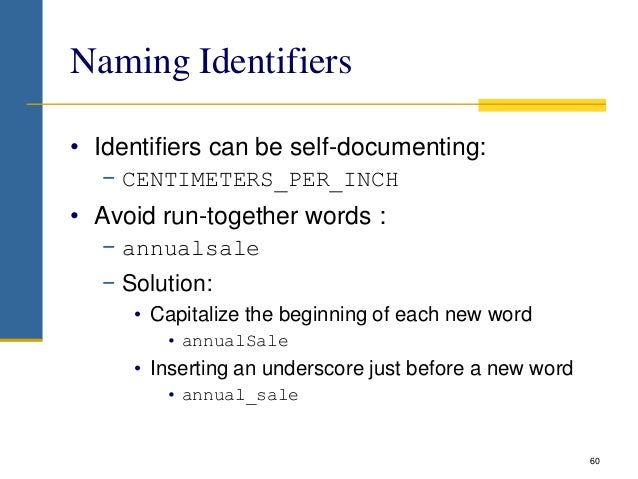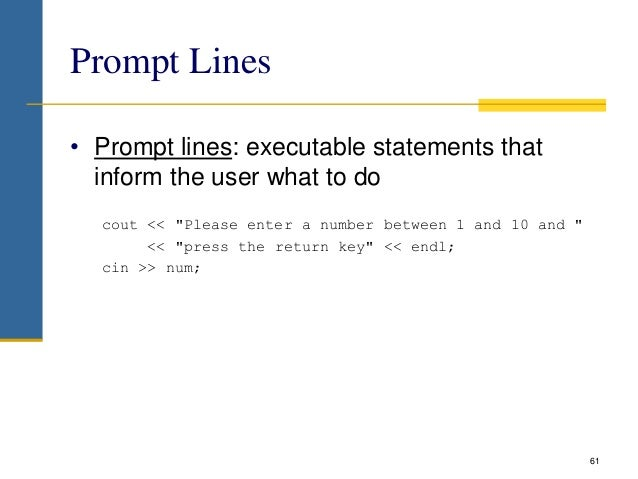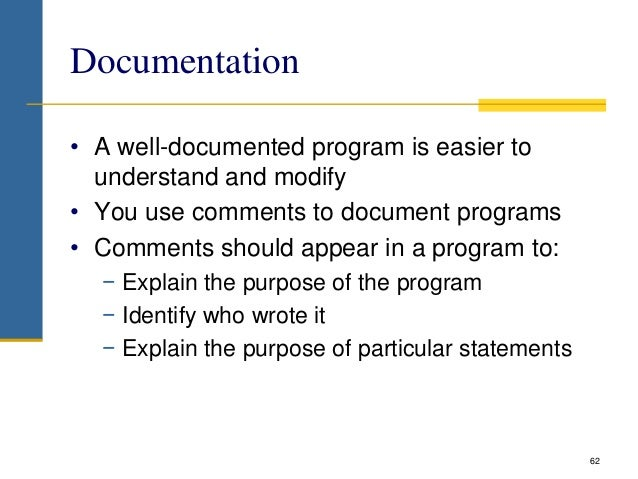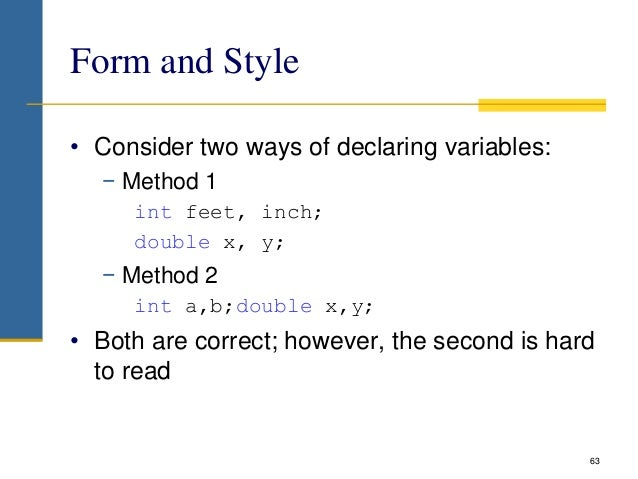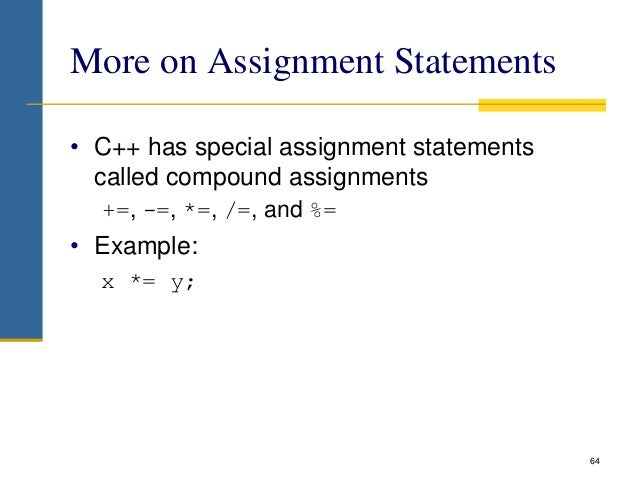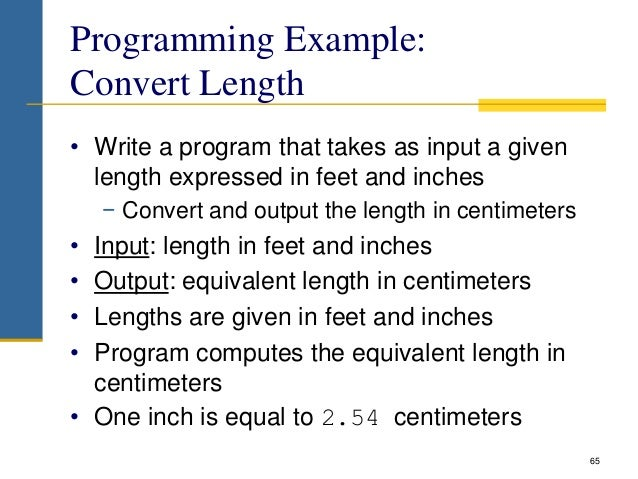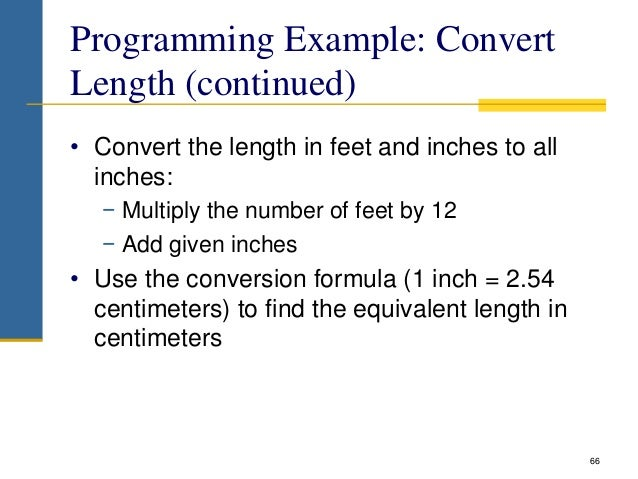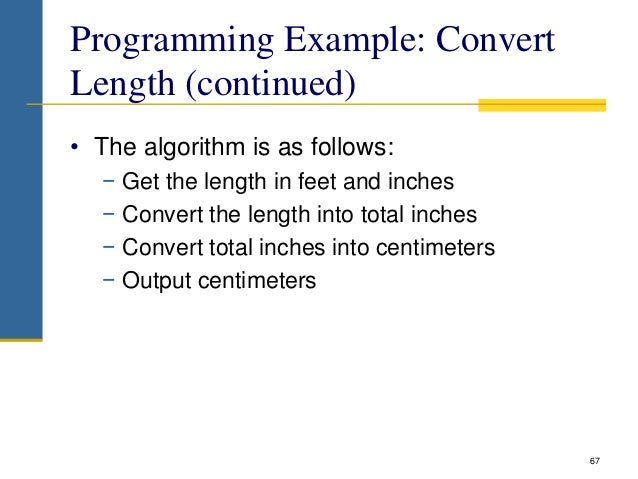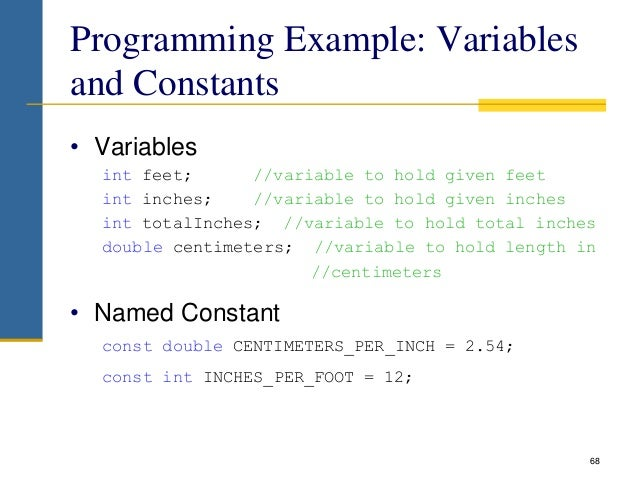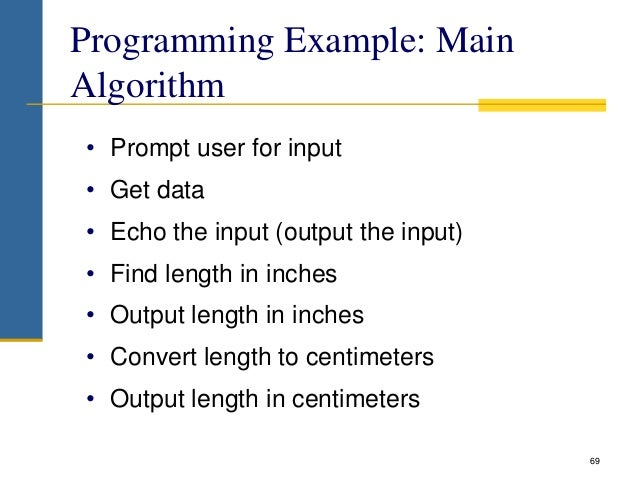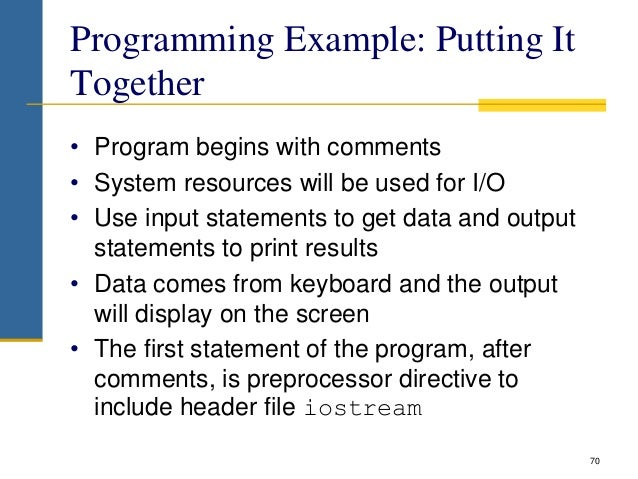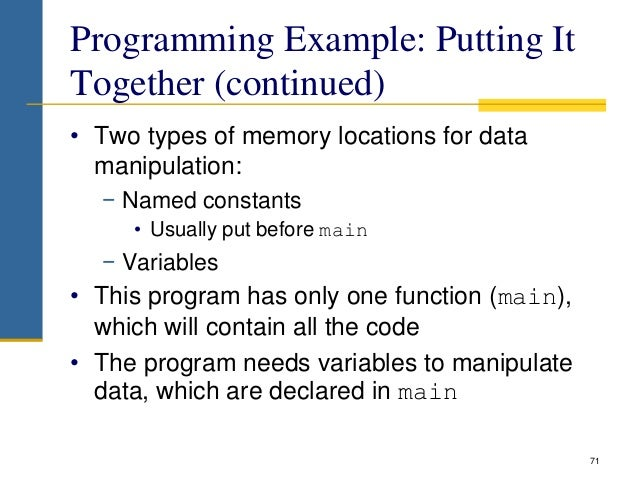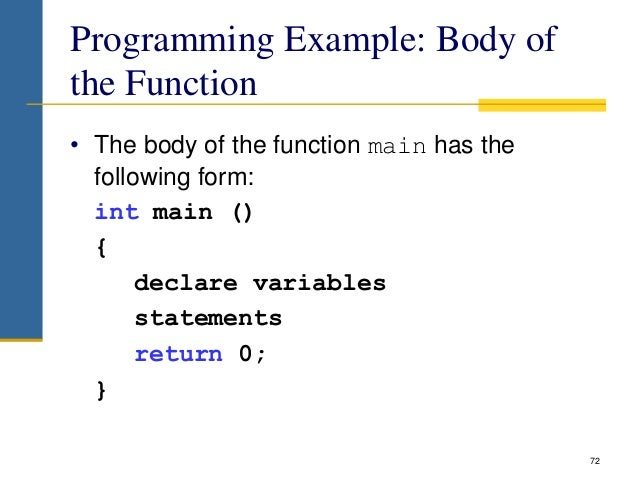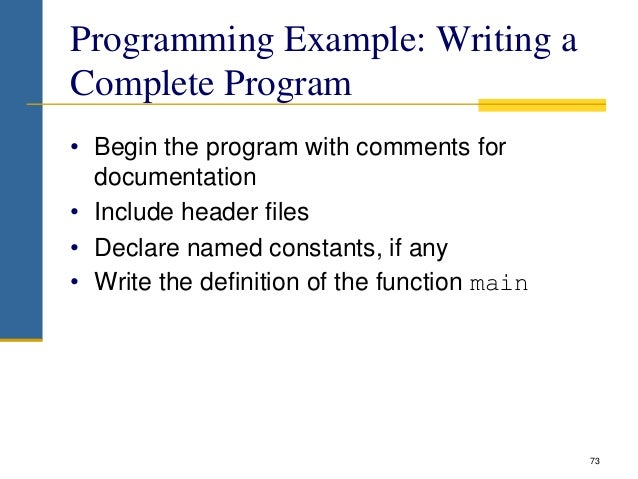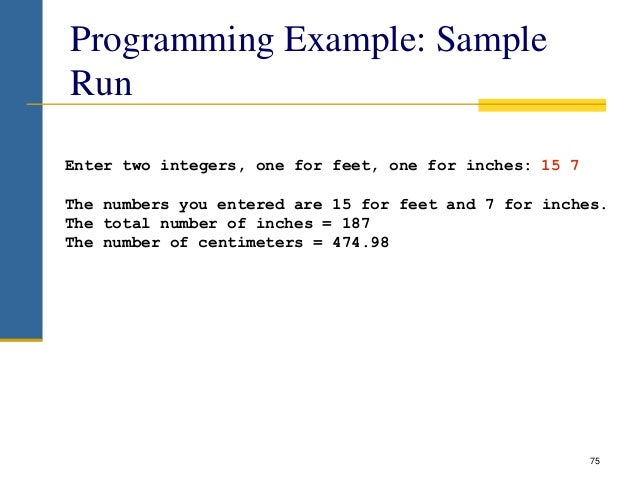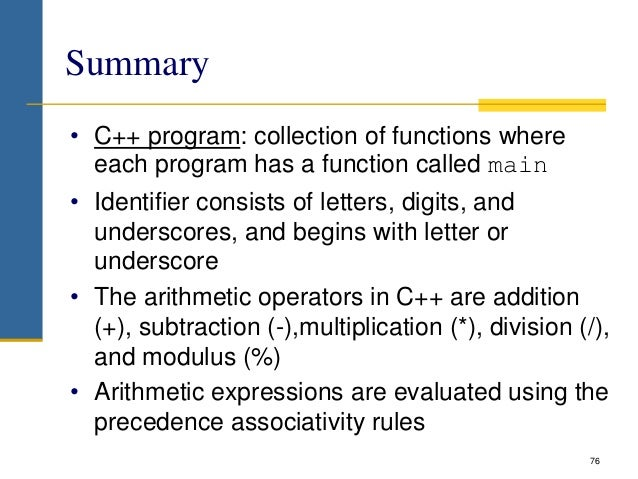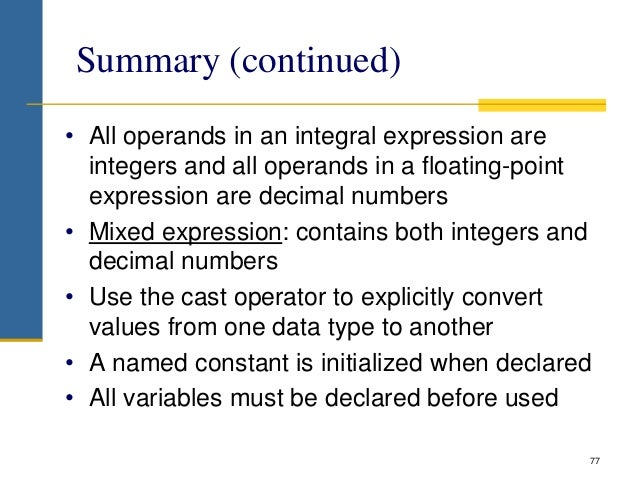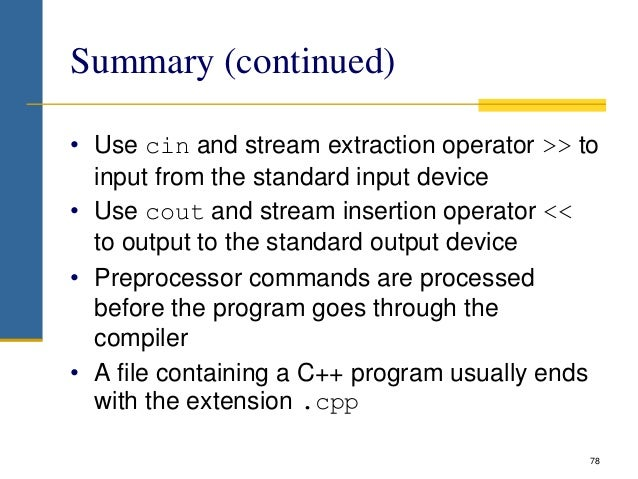Upcoming SlideShare
×
• Full Name
Comment goes here.

Are you sure you want to Yes No• Be the first to comment

### Basics of c++ Programming Language

1. 1. Ahmad Idrees Basics of C++ Programming Language
2. 2. Objectives In this chapter, you will: • Become familiar with the basic components of a C++ program, including functions, special symbols, and identifiers • Explore simple data types • Discover how to use arithmetic operators • Examine how a program evaluates arithmetic expressions 2
3. 3. Objectives (continued) • Learn what an assignment statement is and what it does • Become familiar with the string data type • Discover how to input data into memory using input statements • Become familiar with the use of increment and decrement operators • Examine ways to output results using output statements 3
4. 4. Objectives (continued) • Learn how to use preprocessor directives and why they are necessary • Explore how to properly structure a program, including using comments to document a program • Learn how to write a C++ program 4
5. 5. Structure of a C++ program Source code 1. // my first program in C++ 2. # include <iostream> 3. 4. int main () 5. { 6. std::cout<<“Hello Word!”; 7. } Output Hello Word! 5
6. 6. Components of a C++ program • Line 1: // my first program in C++ • Lines beginning with two slash signs (//) are comments by the programmer and have no effect on the behavior of the program. • Programmers use them to include short explanations or observations concerning the code or program. In this case, it is a brief introductory description of the program. 6
7. 7. Components of a C++ program (Cont.) • Line 2: #include <iostream> • Lines beginning with a hash sign (#) are directives read and interpreted by what is known as the preprocessor. • In this case, the directive #include <iostream>, instructs the preprocessor to include a section of standard C++ code, known as header iostream, that allows to perform standard input and output operations. 7
8. 8. • Line 3: A blank line. • Blank lines have no effect on a program. They simply improve readability. 8 Components of a C++ program (Cont.)
9. 9. • Line 4: int main ( ) • This line initiates the declaration of a function. Essentially, a function is a group of code statements which are given a name: in this case, this gives the name "main" to the group of code statements that follow. • The execution of all C++ programs begins with the main function regardless of where the function is actually located within the code. 9 Components of a C++ program (Cont.)
10. 10. • Line 6: std::cout << "Hello World!"; • This statement has three parts: First, std::cout, which identifies the standard character output device (usually, this is the computer screen). • Second, the insertion operator (<<), which indicates that what follows is inserted into std::cout. • Finally, a sentence within quotes ("Hello world!"), is the content inserted into the standard output. 10 Components of a C++ program (Cont.)
11. 11. The Basics of a C++ Program • Function: collection of statements; when executed, accomplishes something − May be predefined or standard • Syntax: rules that specify which statements (instructions) are legal • Programming language: a set of rules, symbols, and special words • Semantic rule: meaning of the instruction 11
12. 12. Comments • Comments are for the reader, not the compiler • Two types: − Single line // This is a C++ program. It prints the sentence: // Welcome to C++ Programming. − Multiple line /* You can include comments that can occupy several lines. */ 12
13. 13. Special Symbols • Special symbols (Operators) + - * / . ; 13 ? , <= != == >=
14. 14. Reserved Words (Keywords) • Reserved words, keywords, or word symbols − Include: • int • float • double • char • const • void • return 14
15. 15. Identifiers (Variable names) • Consist of letters, digits, and the underscore character ( _ ) • Must begin with a letter or underscore • C++ is case sensitive − NUMBER is not the same as number • Two predefined identifiers are cout and cin • Unlike reserved words, predefined identifiers may be redefined, but it is not a good idea 15
16. 16. Identifiers (continued) • The following are legal identifiers in C++: − First − convert12 − Pay_Rate 16
17. 17. Whitespaces • Every C++ program contains whitespaces − Include blanks, tabs, and newline characters • Used to separate special symbols, reserved words, and identifiers • Proper utilization of whitespaces is important − Can be used to make the program readable 17
18. 18. Simple Data Types Data Type Size in Memory Typical Range (values) Unsigned integer (int) 4 – Bytes 0 to 4294967295 (~10 digits) Signed integer 4 – Bytes -2147483648 to 2147483647 Floating point (float) 4 – Bytes +/- 3.4e +/- 38 (~7 digits) Double (double) 8 – Bytes +/- 1.7e +/- 308 (~15 digits) Character (char) 1 – Byte 0 to 255 Boolean (bool) 1 – Bytes True or false 18  Different compilers may allow different ranges of values
19. 19. int Data Type • Examples: -6728 0 78 +763 • Positive integers do not need a + sign • No commas are used within an integer − Commas are used for separating items in a list 19
20. 20. Floating-Point Data Types − float: represents any real number • Range: -3.4E+38 to 3.4E+38 (four bytes) − double: represents any real number • Range: -1.7E+308 to 1.7E+308 (eight bytes) − On most newer compilers, data types double and long double are same 20
21. 21. Floating-Point Data Types (continued) • Maximum number of significant digits (decimal places) for float values is 7 • Maximum number of significant digits for double is 15 • Precision: maximum number of significant digits − Float values are called single precision − Double values are called double precision 21
22. 22. char Data Type • The smallest integral data type • Used for characters: letters, digits, and special symbols • Each character is enclosed in single quotes − 'A', 'a', '0', '*', '+', '\$', '&' • A blank space is a character and is written ' ', with a space left between the single quotes 22
23. 23. bool Data Type • bool type − Two values: true and false − Manipulate logical (Boolean) expressions • true and false are called logical values • bool, true, and false are reserved words 23
24. 24. Arithmetic Operators and Operator Precedence • C++ arithmetic operators: − + addition − - subtraction − * multiplication − / division − % modulus operator • +, -, *, and / can be used with integral and floating-point data types • Operators can be unary or binary 24
25. 25. Order of Precedence • All operations inside of () are evaluated first • *, /, and % are at the same level of precedence and are evaluated next • + and – have the same level of precedence and are evaluated last • When operators are on the same level − Performed from left to right (associativity) • 3 * 7 - 6 + 2 * 5 / 4 + 6 means (((3 * 7) – 6) + ((2 * 5) / 4 )) + 6 25
26. 26. Expressions • If all operands are integers − Expression is called an integral expression • Yields an integral result • Example: 2 + 3 * 5 • If all operands are floating-point − Expression is called a floating-point expression • Yields a floating-point result • Example: 12.8 * 17.5 - 34.50 26
27. 27. Mixed Expressions • Mixed expression: − Has operands of different data types − Contains integers and floating-point • Examples of mixed expressions: 2 + 3.5 6 / 4 + 3.9 5.4 * 2 – 13.6 + 18 / 2 27
28. 28. Mixed Expressions (continued) • Evaluation rules: − If operator has same types of operands • Evaluated according to the type of the operands − If operator has both types of operands • Integer is changed to floating-point • Operator is evaluated • Result is floating-point − Entire expression is evaluated according to precedence rules 28
29. 29. string Type • Programmer-defined type supplied in ANSI/ISO Standard C++ library • Sequence of zero or more characters • Enclosed in double quotation marks • Null: a string with no characters • Each character has relative position in string − Position of first character is 0 • Length of a string is number of characters in it − Example: length of "William Jacob" is 13 29
30. 30. Input • Data must be loaded into main memory before it can be manipulated • Storing data in memory is a two-step process: − Instruct computer to allocate memory − Include statements to put data into memory 30
31. 31. Declaring & Initializing Variables • Variables can be initialized when declared: int first=13, second=10; char ch=' '; double x=12.6; • All variables must be initialized before they are used − But not necessarily during declaration 31
32. 32. Putting Data into Variables • Ways to place data into a variable: − Use C++’s assignment statement − Use input (read) statements 32
33. 33. Allocating Memory with Constants and Variables • Variable: memory location whose content may change during execution • The syntax to declare a named constant is: 33
34. 34. Allocating Memory with Constants and Variables (continued) • Named constant: memory location whose content can’t change during execution • The syntax to declare a named constant is: • In C++, const is a reserved word 34
35. 35. Assignment Statement • The assignment statement takes the form: • Expression is evaluated and its value is assigned to the variable on the left side • In C++, = is called the assignment operator 35
36. 36. Assignment Statement (continued) 36
37. 37. Saving and Using the Value of an Expression • To save the value of an expression: − Declare a variable of the appropriate data type − Assign the value of the expression to the variable that was declared • Use the assignment statement • Wherever the value of the expression is needed, use the variable holding the value 37
38. 38. Input (Read) Statement • cin is used with >> to gather input • The stream extraction operator is >> • For example, if miles is a double variable cin >> miles; − Causes computer to get a value of type double − Places it in the variable miles 38
39. 39. Input (Read) Statement (continued) • Using more than one variable in cin allows more than one value to be read at a time • For example, if feet and inches are variables of type int, a statement such as: cin >> feet >> inches; − Inputs two integers from the keyboard − Places them in variables feet and inches respectively 39
40. 40. Input (Read) Statement (continued) 40
41. 41. Variable Initialization • There are two ways to initialize a variable: int feet; − By using the assignment statement feet = 35; − By using a read statement cin >> feet; 41
42. 42. Increment & Decrement Operators • Increment operator: increment variable by 1 − Pre-increment: ++variable − Post-increment: variable++ • Decrement operator: decrement variable by 1 − Pre-decrement: --variable − Post-decrement: variable— • What is the difference between the following? 42 x = 5; y = ++x; x = 5; y = x++;
43. 43. Output • The syntax of cout and << is: − Called an output statement • The stream insertion operator is << • Expression evaluated and its value is printed at the current cursor position on the screen 43
44. 44. Output (continued) • A manipulator is used to format the output − Example: endl causes insertion point to move to beginning of next line 44
45. 45. Output (continued) • The new line character is 'n' − May appear anywhere in the string cout << "Hello there."; cout << "My name is James."; • Output: Hello there.My name is James. cout << "Hello there.n"; cout << "My name is James."; • Output : Hello there. My name is James. 45
46. 46. Output (continued) 46
47. 47. Preprocessor Directives • C++ has a small number of operations • Many functions and symbols needed to run a C++ program are provided as collection of libraries • Every library has a name and is referred to by a header file • Preprocessor directives are commands supplied to the preprocessor • All preprocessor commands begin with # • No semicolon at the end of these commands 47
48. 48. Preprocessor Directives (continued) • Syntax to include a header file: • For example: #include <iostream> − Causes the preprocessor to include the header file iostream in the program 48
49. 49. namespace and Using cin and cout in a Program • cin and cout are declared in the header file iostream, but within std namespace • To use cin and cout in a program, use the following two statements: #include <iostream> using namespace std; 49
50. 50. Using the string Data Type in a Program • To use the string type, you need to access its definition from the header file string • Include the following preprocessor directive: #include <string> 50
51. 51. Creating a C++ Program • C++ program has two parts: − Preprocessor directives − The program • Preprocessor directives and program statements constitute C++ source code (.cpp) • Compiler generates object code (.obj) • Executable code is produced and saved in a file with the file extension .exe 51
52. 52. Creating a C++ Program (continued) • A C++ program is a collection of functions, one of which is the function main • The first line of the function main is called the heading of the function: int main() • The statements enclosed between the curly braces ({ and }) form the body of the function − Contains two types of statements: • Declaration statements • Executable statements 52
53. 53. 53
54. 54. Creating a C++ Program (continued) Sample Run: Line 9: firstNum = 18 Line 10: Enter an integer: 15 Line 13: secondNum = 15 Line 15: The new value of firstNum = 60 54
55. 55. Program Style and Form • Every C++ program has a function main • It must also follow the syntax rules • Other rules serve the purpose of giving precise meaning to the language 55
56. 56. Syntax • Errors in syntax are found in compilation int x; //Line 1 int y //Line 2: error double z; //Line 3 y = w + x; //Line 4: error 56
57. 57. Use of Blanks • In C++, you use one or more blanks to separate numbers when data is input • Used to separate reserved words and identifiers from each other and from other symbols • Must never appear within a reserved word or identifier 57
58. 58. Use of Semicolons, Brackets, and Commas • All C++ statements end with a semicolon − Also called a statement terminator • { and } are not C++ statements • Commas separate items in a list 58
59. 59. Semantics • Possible to remove all syntax errors in a program and still not have it run • Even if it runs, it may still not do what you meant it to do • For example, 2 + 3 * 5 and (2 + 3) * 5 are both syntactically correct expressions, but have different meanings 59
60. 60. Naming Identifiers • Identifiers can be self-documenting: − CENTIMETERS_PER_INCH • Avoid run-together words : − annualsale − Solution: • Capitalize the beginning of each new word • annualSale • Inserting an underscore just before a new word • annual_sale 60
61. 61. Prompt Lines • Prompt lines: executable statements that inform the user what to do cout << "Please enter a number between 1 and 10 and " << "press the return key" << endl; cin >> num; 61
62. 62. Documentation • A well-documented program is easier to understand and modify • You use comments to document programs • Comments should appear in a program to: − Explain the purpose of the program − Identify who wrote it − Explain the purpose of particular statements 62
63. 63. Form and Style • Consider two ways of declaring variables: − Method 1 int feet, inch; double x, y; − Method 2 int a,b;double x,y; • Both are correct; however, the second is hard to read 63
64. 64. More on Assignment Statements • C++ has special assignment statements called compound assignments +=, -=, *=, /=, and %= • Example: x *= y; 64
65. 65. Programming Example: Convert Length • Write a program that takes as input a given length expressed in feet and inches − Convert and output the length in centimeters • Input: length in feet and inches • Output: equivalent length in centimeters • Lengths are given in feet and inches • Program computes the equivalent length in centimeters • One inch is equal to 2.54 centimeters 65
66. 66. Programming Example: Convert Length (continued) • Convert the length in feet and inches to all inches: − Multiply the number of feet by 12 − Add given inches • Use the conversion formula (1 inch = 2.54 centimeters) to find the equivalent length in centimeters 66
67. 67. Programming Example: Convert Length (continued) • The algorithm is as follows: − Get the length in feet and inches − Convert the length into total inches − Convert total inches into centimeters − Output centimeters 67
68. 68. Programming Example: Variables and Constants • Variables int feet; //variable to hold given feet int inches; //variable to hold given inches int totalInches; //variable to hold total inches double centimeters; //variable to hold length in //centimeters • Named Constant const double CENTIMETERS_PER_INCH = 2.54; const int INCHES_PER_FOOT = 12; 68
69. 69. Programming Example: Main Algorithm • Prompt user for input • Get data • Echo the input (output the input) • Find length in inches • Output length in inches • Convert length to centimeters • Output length in centimeters 69
70. 70. Programming Example: Putting It Together • Program begins with comments • System resources will be used for I/O • Use input statements to get data and output statements to print results • Data comes from keyboard and the output will display on the screen • The first statement of the program, after comments, is preprocessor directive to include header file iostream 70
71. 71. Programming Example: Putting It Together (continued) • Two types of memory locations for data manipulation: − Named constants • Usually put before main − Variables • This program has only one function (main), which will contain all the code • The program needs variables to manipulate data, which are declared in main 71
72. 72. Programming Example: Body of the Function • The body of the function main has the following form: int main () { declare variables statements return 0; } 72
73. 73. Programming Example: Writing a Complete Program • Begin the program with comments for documentation • Include header files • Declare named constants, if any • Write the definition of the function main 73
74. 74. 74
75. 75. Programming Example: Sample Run 75 Enter two integers, one for feet, one for inches: 15 7 The numbers you entered are 15 for feet and 7 for inches. The total number of inches = 187 The number of centimeters = 474.98
76. 76. Summary • C++ program: collection of functions where each program has a function called main • Identifier consists of letters, digits, and underscores, and begins with letter or underscore • The arithmetic operators in C++ are addition (+), subtraction (-),multiplication (*), division (/), and modulus (%) • Arithmetic expressions are evaluated using the precedence associativity rules 76
77. 77. Summary (continued) • All operands in an integral expression are integers and all operands in a floating-point expression are decimal numbers • Mixed expression: contains both integers and decimal numbers • Use the cast operator to explicitly convert values from one data type to another • A named constant is initialized when declared • All variables must be declared before used 77
78. 78. Summary (continued) • Use cin and stream extraction operator >> to input from the standard input device • Use cout and stream insertion operator << to output to the standard output device • Preprocessor commands are processed before the program goes through the compiler • A file containing a C++ program usually ends with the extension .cpp 78

### Be the first to comment

•#### jackkim22

Jul. 27, 2019
•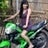#### ErneflorManaay

Aug. 10, 2019
•#### NeerajKumar1694

Aug. 26, 2019
•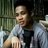#### MarkBastasa

Aug. 28, 2019
•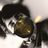#### Maaz21

Oct. 17, 2019
•#### musaAslam1

Oct. 27, 2019
•Dec. 11, 2019
•#### YousifAbdulBasit

Dec. 18, 2019
•#### TheodoraYendao

Feb. 2, 2020
•#### AFTABALI118

Feb. 10, 2020
•#### suraganigeetha

Apr. 8, 2020
•Apr. 30, 2020
•#### santanuic012

May. 1, 2020
•May. 26, 2020
•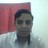#### return001

Aug. 20, 2020
•#### SaiSresta

Sep. 15, 2020
•Feb. 18, 2021
•#### MojtabaSoli

Mar. 2, 2021
•#### JohnMaliwa

May. 8, 2021
•#### KannanPBinu

Jun. 27, 2021

Total views

39,447

On Slideshare

0

From embeds

0

Number of embeds

986

5,758

Shares

0